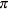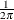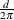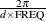The SPECTRA Procedure

Transforming Frequencies

The variable FREQ in the data set created by the SPECTRA procedure ranges from 0 to. Sometimes it is preferable to express frequencies in cycles per observation period, which is equal toFREQ.

To express frequencies in cycles per unit time (for example, in cycles per year), multiply FREQ by, where d is the number of observations per unit of time. For example, for monthly data, if the desired time unit is years then d is 12. The period of the cycle is, which ranges fromto infinity.